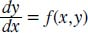NextPrevious

# What are some first-order differential equations?

A first-order differential equation is one involving the unknown function y, its derivative y', and the variable x. As seen above, these types of equations are usually referred to as explicit differential equations.

There are several types of first-order differential equations, including separable, Bernoulli, linear, and homogeneous (for explanations of the last two, see below). A first-order differential equation takes the form:Close

This is a web preview of the "The Handy Math Answer Book" app. Many features only work on your mobile device. If you like what you see, we hope you will consider buying. Get the App